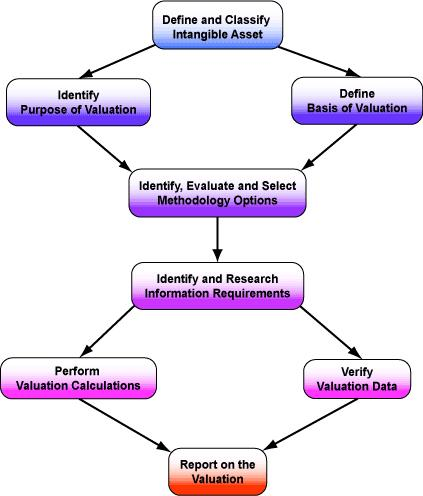# THE VALUATION PROCESS

The valuation process is represented by the diagram below. Each of the sections of the process are covered by following the links at the top of this page.Before launching into any valuation, it is important to be aware of the limitations and usefulness of valuations and the different approaches that can be used for assessing value.
Understanding limitations is exceptionally important and has been emphasised in any balanced assessment of the valuation process. For example Aswath Damodaran in his work has referred to the Myths and Truths of Valuation.
Myth 1: A valuation is an objective search for "true" value.
• Truth 1.1: All valuations are biased. The only questions are how much and in which direction.
• Truth 1.2: The direction and magnitude of the bias in a valuation is directly proportional to who pays and how much is paid.
Myth 2: A good valuation provides a precise estimate of value
• Truth 2.1: There are no precise valuations.
• Truth 2.2: The payoff to valuation is greatest when valuation is least precise.
Myth 3: The more quantitative a model, the better the valuation.
• Truth 3.1: One's understanding of a valuation model is inversely proportional to the number of inputs required for the model.
• Truth 3.2: Simpler valuation models do much better than complex ones.
The use of valuation models in investment decisions (i.e. in decisions on which assets are under valued and which are over valued) is based on:
• a perception that markets are inefficient and make mistakes in assessing value and
• an assumption about how and when these inefficiencies will get corrected.
In an efficient market, the market price is the best estimate of value. The purpose of any valuation model is then the justification of this value.